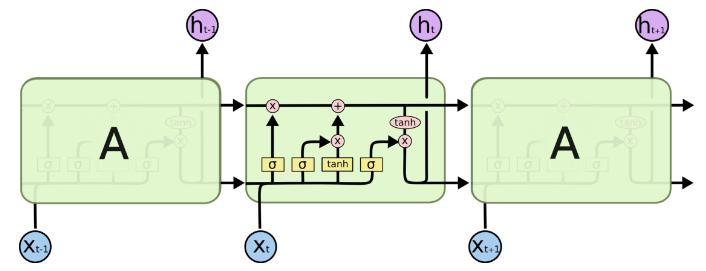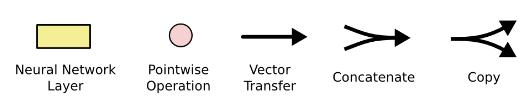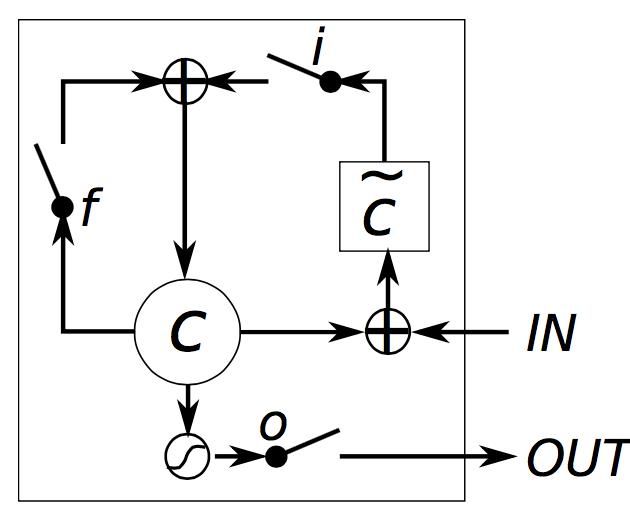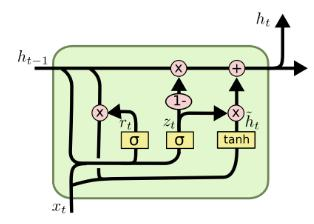# 递归神经网络## 1 RNN

• $$\nabla_{o_i^{(t)}}L=\nabla_{o_i^{(t)}} L^{(t)}=-\nabla_{o_i^{(t)}}\log \frac{\exp(o_{y^{(t)}}^{(t)})}{\sum_j\exp(o_j^{(t)})}=\hat{y}_i^{(t)}-\mathbf{l}{i=y^{(t)}}$$

• 向量表示： $$\nabla_{o^{(t)}}L=\hat{\mathbf{y}}^{(t)}-\mathbf{y}^{(t)}$$ 其中$\mathbf{y}^{(t)}$是由标量标签扩展的one-hot向量。即总的损失对任意一个时刻的输出的导数都可以表示成上述形式。

• 对最后一个时间$\tau$的导数： $$\nabla_{h^{(\tau)}}L=V^T\nabla_{o^{(\tau)}}L^{(t)}$$

• 对于任意的$t<\tau$，$h^{(t)}$接收两个方向回传的梯度：$h^{(t+1)}$和$o^{(t)}$。 \begin{align}\nabla_{h^{(t)}}L &=\left(\frac{\partial o^{(t)}}{\partial h^{(t)}}\right)^T\nabla_{o^{(t)}}L+\left(\frac{\partial h^{(t+1)}}{\partial h^{(t)}}\right)^T\nabla_{h^{(t+1)}}L \cr &= V^T\nabla_{o^{(t)}}L + W^T\mathrm{diag}(1-(h^{(t+1)})^2)(\nabla_{h^{(t+1)}}L)\end{align}

• 对参数的求导，因为所有时间步共享参数，因此需要对所有时间步累加导数。 $$\begin{equation} \begin{split} \nabla_c L &= \sum_t \nabla_{o^{(t)}} L \cr \nabla_b L&=\sum_t \mathrm{diag}(1-(h^{(t)})^2)\nabla_{h^{(t)}}L \cr \nabla_V L &= \sum_t (\nabla_{o^{(t)}}L) (h^{(t)})^T \cr \nabla_W L &= \sum_t \mathrm{diag}(1-(h^{(t)})^2)(\nabla_{h^{(t)}}L) (h^{(t-1)})^T \cr \nabla_U L &= \sum_t \mathrm{diag}(1-(h^{(t)})^2)(\nabla_{h^{(t)}}L) (x^{(t)})^T \end{split} \end{equation}$$

• 传统的RNN会有梯度消失问题，这是因为误差项累积相乘，也就是不能保持长期记忆。因此需要引入一些控制门缓解梯度消失，这样做会使得梯度误差项变成相加。

## 2 LSTM

LSTM的提出是希望改善RNN缺少长期记忆的缺点。RNN当时间步达到一定长度时，模型很可能会忘掉之前的记忆。首先来看一张形象的LSTM模型图：RNN时LSTM的特殊情况：当输入门全1，遗忘门全0（总是抛弃过去的记忆），输出门全1时，就是一个RNN。

## 3 GRU

GRU将LSTM的输入门和遗忘门合并成一个更新门$z$，并且将细胞状态和输出状态合并。